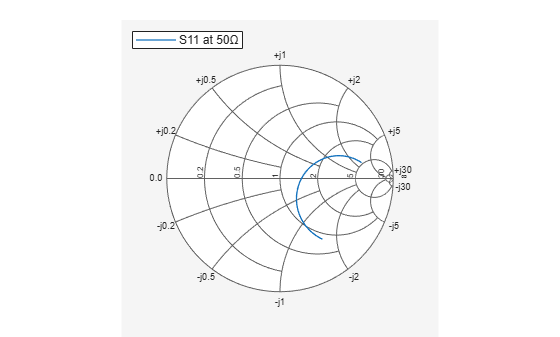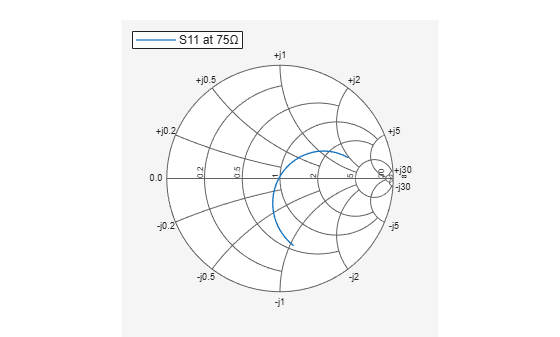# replace

Remove current data and add new data to Smith chart

## Syntax

``replace(plot,data)``
``replace(plot,frequency,data)``

## Description

````replace(plot,data)` removes all current data from a Smith chart, `plot`, and adds new data to the Smith chart.```
````replace(plot,frequency,data)` removes all current data and adds new data to the Smith chart based on multiple data sets containing frequencies corresponding to columns of the data matrix.```

## Examples

collapse all

Plot the reflection coefficients of a dipole antenna.

Create a strip dipole antenna on the Y-Z plane. Calculate the complex S-parameters of the dipole antenna from 60 MHz to 90 MHz, with an interval of 150 kHz.

Plot S11 on a Smith chart for a reference impedance of 50 ohm.

```d = dipole; freq = linspace(60e6,90e6,200); s_50 = sparameters(d,freq,50); hg = smithplot(s_50,[1,1]); hg.LegendLabels = 'S11 at 50#ohm'; ```Find S11 for a new impedance of 75 ohm. Replace the old S11 by the new S11 on the existing Smith chart.

```s_75 = sparameters(d,freq,75); gamma = rfparam(s_75,1,1); replace(hg,gamma); hg.LegendLabels = 'S11 at 75#ohm';```## Input Arguments

collapse all

Smith chart handle, specified as a plot handle. If the handle of the Smith chart is not retained during creation, use ```p = smithplot('gco')```.

Input data, specified as a complex vector or complex matrix.

For a matrix D, the columns of D are independent datasets. For N-by-D arrays, dimensions 2 and greater are independent datasets.

Data Types: `double`
Complex Number Support: Yes

Frequency data, specified as a real vector.

Data Types: `double`

## Version History

Introduced in R2017b